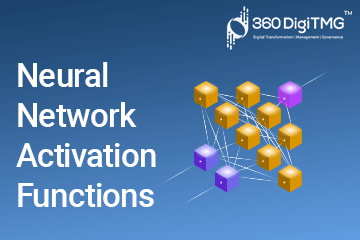Home / Blog / Artificial Intelligence / Optimizers

# Optimizers

• July 13, 2023
• 4077
• 6### Meet the Author : Mr. Bharani Kumar

Bharani Kumar Depuru is a well known IT personality from Hyderabad. He is the Founder and Director of Innodatatics Pvt Ltd and 360DigiTMG. Bharani Kumar is an IIT and ISB alumni with more than 17 years of experience, he held prominent positions in the IT elites like HSBC, ITC Infotech, Infosys, and Deloitte. He is a prevalent IT consultant specializing in Industrial Revolution 4.0 implementation, Data Analytics practice setup, Artificial Intelligence, Big Data Analytics, Industrial IoT, Business Intelligence and Business Management. Bharani Kumar is also the chief trainer at 360DigiTMG with more than Ten years of experience and has been making the IT transition journey easy for his students. 360DigiTMG is at the forefront of delivering quality education, thereby bridging the gap between academia and industry.

Table of Content

Whether selecting the parameters, creating a model, or training a model, we are always interested in a procedure that is completed swiftly. Unfortunately, it takes a long time to train a model on complicated data.

One method for updating the weight of the network is backpropagation, which helps the model perform better by reducing loss and producing more accurate predictions. By eliminating the issues that may result in their being stuck in various locations, the gradient descent is intended to descend more quickly to the minima. For us to swiftly converge to the minima, certain algorithms offer the optimum learning rate. These programmes are referred to as optimizers. It's crucial to avoid the following surfaces when descending a hill.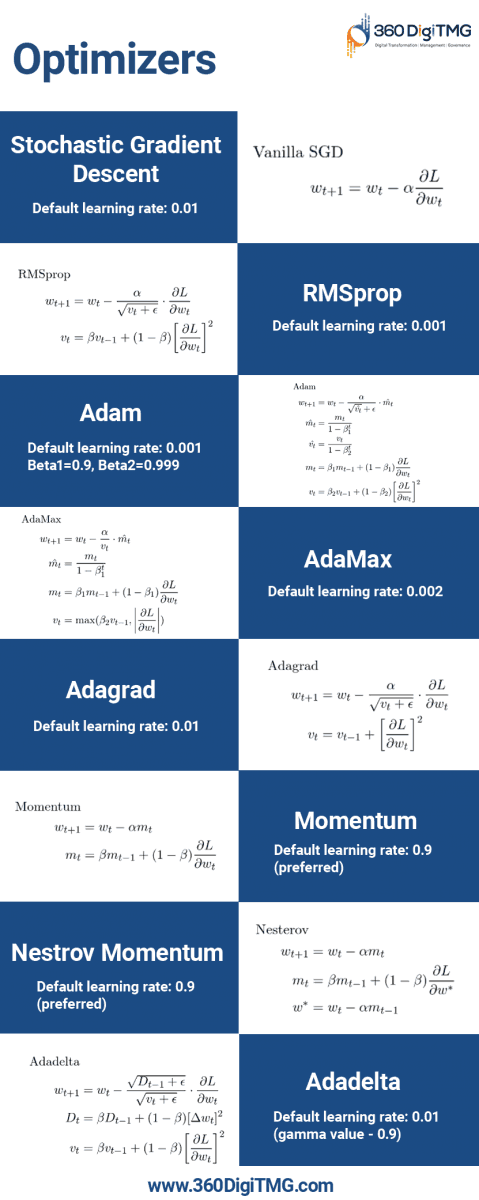Minimum: It is a point on the error surface where the gradient is zero, but if there is a movement in any direction then it will lead us to move upwards.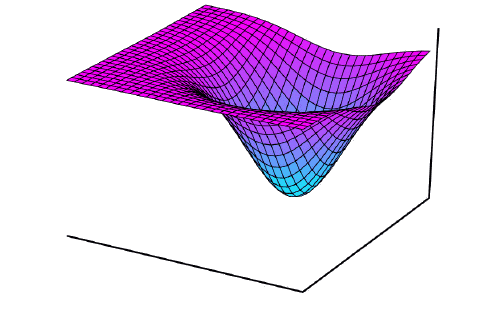Plateau: It's a level area, so no matter which direction we travel, we're never moving up or down. When a point is on the flat surface, the gradient is 0.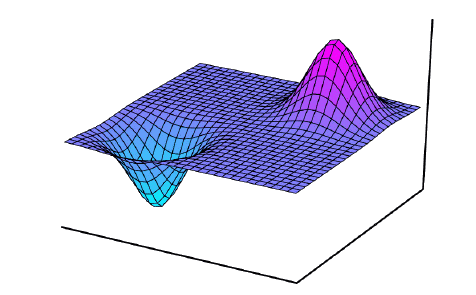Saddle: It is an error surface where a moment in one or more axes will increase the error, or movement in one or more axes decreases the error.It is crucial for the gradient descent method to avoid these locations. On the other hand, certain slopes are noisy and not at all near to zero. To converge minimum, this noisy gradient travels in a zigzag pattern.

We will employ optimizers to assist us get to the minima rapidly and noise-free in order to avoid these issues. The key optimizers that will help the neural network perform better and learn more quickly are listed below.

• ## Stochastic Gradient Descent with Moment

Stochastic gradient descent picks the data point randomly from a dataset at each iteration which will reduce the computation. This gradient descent update the current weights by multiplying a constant value called learning rate, .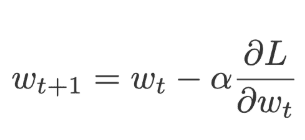When using SGD with momentum, we calculate the weight's change for each iteration and then add a little portion of its change from the prior iteration. A momentum(m) takes the place of the present weights. where momentum is the pace at which the weights from the past and the present are changing. M is initially initialised to 0.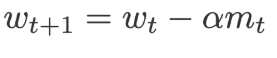Where,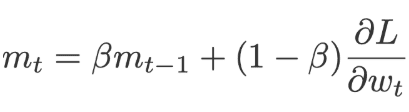β=0.9 (scaling factor)

Adagrad is referred to as an adaptable gradient because, as the name implies, the method adjusts the gradient's size at each weight. It is used to the learning rate, which is calculated by dividing the squared gradients of the past and present cumulatively(v).

The value contributed to the total is always positive since each iteration squares the gradients before adding them. In order to ensure that we will never see a value that has been split by zero, a floating-point number has also been added to the variable "v." In Keras, this is referred to as a Fuzz factor.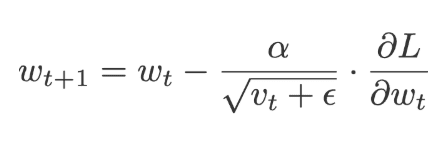Where,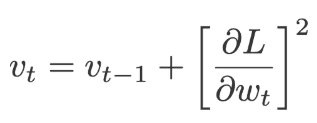The default values of α=0.01 and ε=10-7

• ## RMSprop

Root means square prop is the full term given to the RMSprop. RMSprop employs a parameter to control how to remember, whereas Adadelta operates along similar lines. RMSprop takes into account the exponential moving average of the gradients as opposed to the Aadagrad, which takes the cumulative value of squared gradients.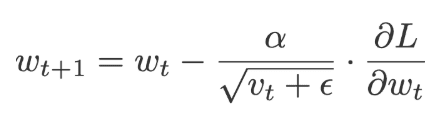Where,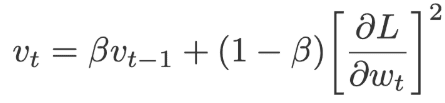The default values for:

α=0.01, β= 0.9 (recommended) and ε= 10-6

Initialising v and D to 0 will set their values.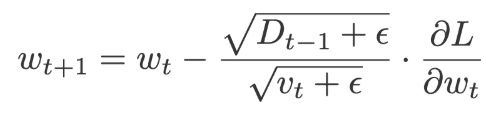Where,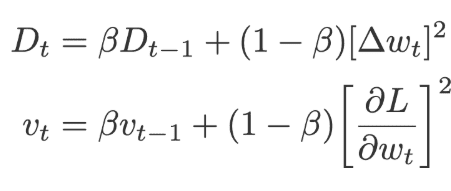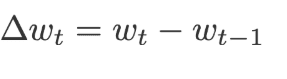The default values of ε= 10-6, β=0.95, α=0.01

Adam, also known as an adaptive moment estimate, is created by fusing momentum and RMSprop. Adam increases the gradient by adding the component m, or the gradients' exponential moving average. By dividing the learning rate () by the square root of the exponential moving average of squared gradients (v), the learning rate () is added.

To fix the bias, apply the following equations: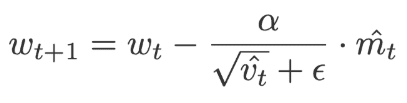Where,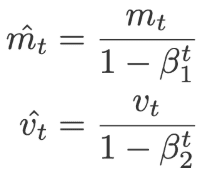And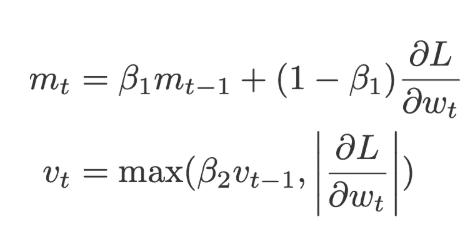Where m, v is initialized to 0 along with

α=0.001, β1=0.9 and β2=0.99 and ε= 10-8

Adamas is a subtype of Adam; models with embeddings frequently employ these optimizers. Here, the maximum function is approximated by using the exponential moving average of the gradients (m) and the exponential moving average of the old p-norm of the gradients (v), respectively. For bias correction, apply the subsequent equation.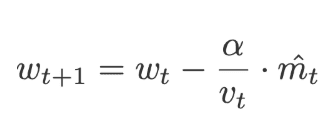Where,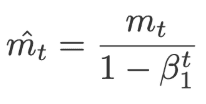AndWhere m and v are initialized to 0 along with

α=0.002, β11=0.9, β2=0.999

• ## Nesterov Momentum

We can move past information with the aid of momentum and train the network. However, it will eventually reach us via Nesterov momentum.

The fundamental concept is that we may employ gradients at a position where we could be in the future rather than at a spot where we are right now.

It is comparable to Momentum, which uses an exponential moving average with an initial value of 0.

It will use the prior velocity to update the current weights.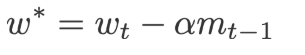In order to calculate the current weights (w) and the exponential moving average of squared gradients (v), forward propagation is utilised to compute this value's gradients for the same weights.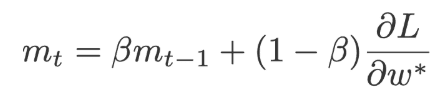Where β=0.9 and α=0.9 preferred.

Click here to learn Data Science Course, Data Science Course in Hyderabad, Data Science Course in Bangalore

### Data Science Training Institutes in Other Locations